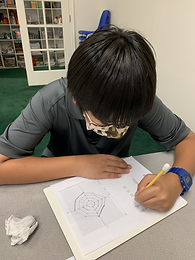Ms. Kayla

Target 1​

Review

Number Operation

:

Computation

2:

Identify the steps necessary to avoid making easy computational errors when adding decimal numbers.

3:

When calculating, align the decimal points in each number, then add hundredths, tenths, ones, and tens.

4:

Apply regrouping strategies wherever necessary when adding decimal numbers.

5th

Vocabulary:

Place Value, Hundredths, tenths, Tens, Decimal, Sum, Regroup

Activities:

Students practiced computation using the standard algorithm to find the sum and difference of decimals.

Students demonstrated understanding of decimal alignment by correctly aligning decimal numbers to complete their computation.

Students also demonstrated understanding of regrouping.Home Exploration

Guiding Questions:Absent Students:

Target 2

:

5th

Vocabulary:

Coordinate pairs, Coordinate plane/grid, X-axis, Y-axis, Negative, Positive

Activities:

Students plotted point on a coordinate plane/grid to create an image.

Students used all 4 quadrants on the coordinate plane to plot the coordinate pairs.

Students demonstrated understanding of coordinate pairs (x,y).Home Exploration

Guiding Questions:Target 3

:

Vocabulary:

Activities:Home Exploration